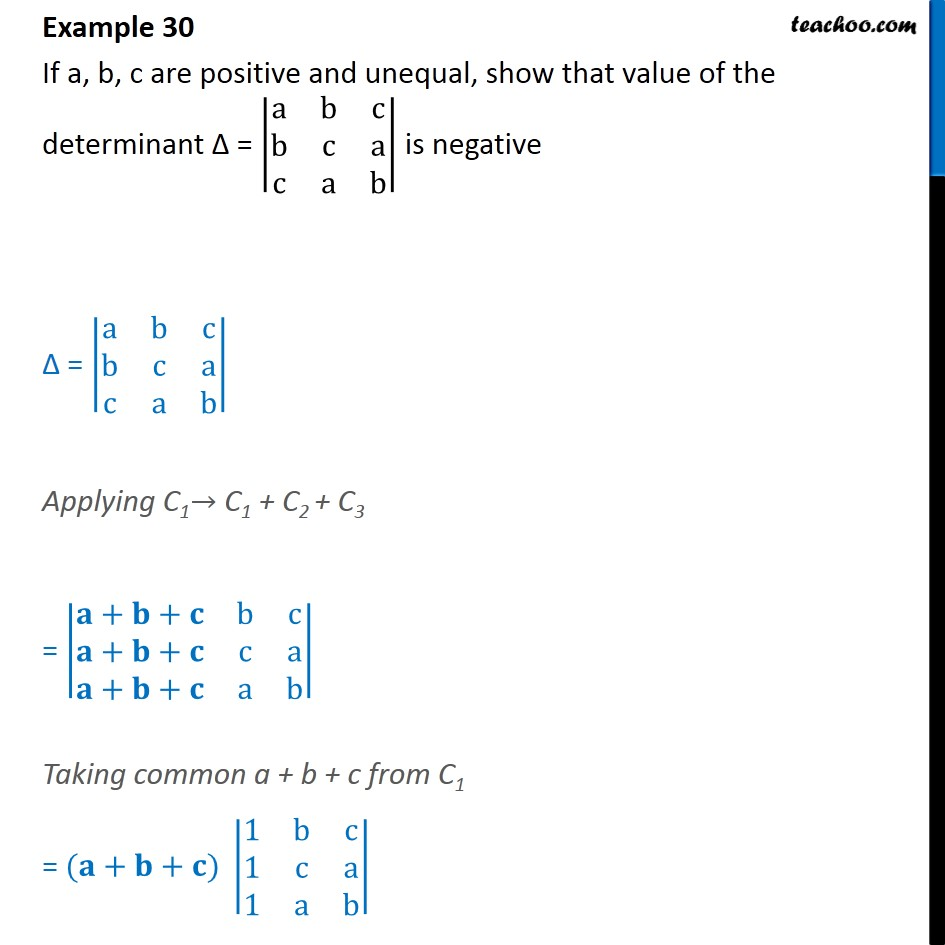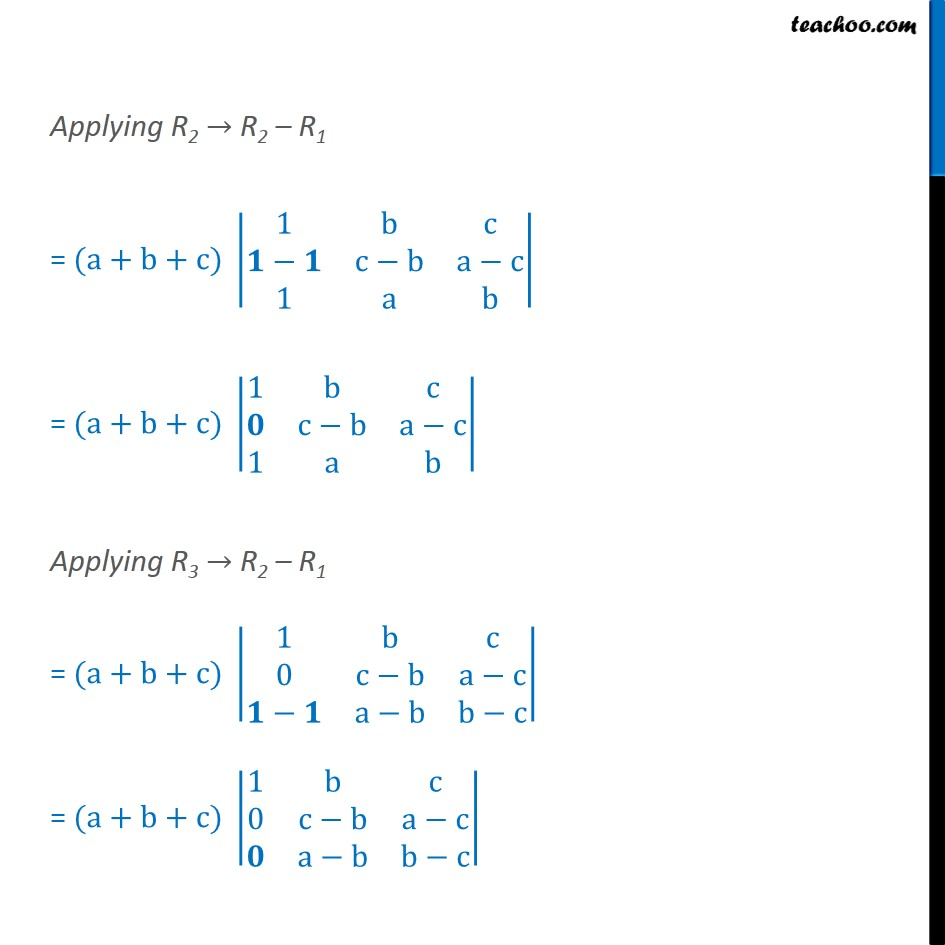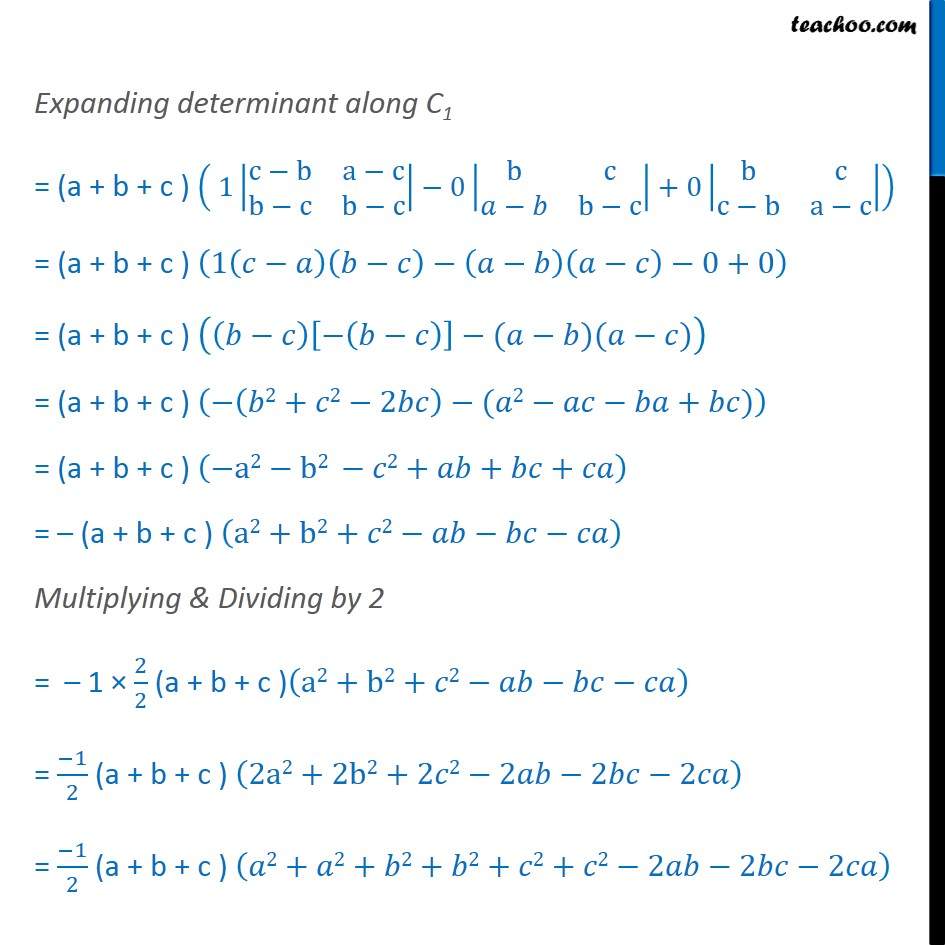Examples

Chapter 4 Class 12 Determinants
Serial order wiseLearn in your speed, with individual attention - Teachoo Maths 1-on-1 Class

### Transcript

Question 12 If a, b, c are positive and unequal, show that value of the determinant Δ = a﷮b﷮c﷮b﷮c﷮a﷮c﷮a﷮b﷯﷯ is negative Δ = a﷮b﷮c﷮b﷮c﷮a﷮c﷮a﷮b﷯﷯ Applying C1→ C1 + C2 + C3 = 𝐚+𝐛+𝐜﷮b﷮c﷮𝐚+𝐛+𝐜﷮c﷮a﷮𝐚+𝐛+𝐜﷮a﷮b﷯﷯ Taking common a + b + c from C1 = (𝐚+𝐛+𝐜) 1﷮b﷮c﷮1﷮c﷮a﷮1﷮a﷮b﷯﷯ Applying R2 → R2 – R1 = (a+b+c) 1﷮b﷮c﷮𝟏−𝟏﷮c−b﷮a−c﷮1﷮a﷮b﷯﷯ = (a+b+c) 1﷮b﷮c﷮𝟎﷮c−b﷮a−c﷮1﷮a﷮b﷯﷯ Applying R3 → R2 – R1 = (a+b+c) 1﷮b﷮c﷮0﷮c−b﷮a−c﷮𝟏−𝟏﷮a−b﷮b−c﷯﷯ = (a+b+c) 1﷮b﷮c﷮0﷮c−b﷮a−c﷮𝟎﷮a−b﷮b−c﷯﷯ Expanding determinant along C1 = (a + b + c ) 1 c−b﷮a−c﷮b−c﷮b−c﷯﷯−0 b﷮c﷮𝑎−𝑏﷮b−c﷯﷯+0 b﷮c﷮c−b﷮a−c﷯﷯﷯ = (a + b + c ) 1 𝑐−𝑎﷯ 𝑏−𝑐﷯− 𝑎−𝑏﷯ 𝑎−𝑐﷯−0+0﷯ = (a + b + c ) 𝑏−𝑐﷯ − 𝑏−𝑐﷯﷯−(𝑎−𝑏)(𝑎−𝑐)﷯ = (a + b + c ) − 𝑏2+𝑐2−2𝑏𝑐﷯−(𝑎2−𝑎𝑐−𝑏𝑎+𝑏𝑐)﷯ = (a + b + c ) −a2−b2 −𝑐2+𝑎𝑏+𝑏𝑐+𝑐𝑎﷯ = – (a + b + c ) a2+b2+𝑐2−𝑎𝑏−𝑏𝑐−𝑐𝑎﷯ Multiplying & Dividing by 2 = – 1 × 2﷮2﷯ (a + b + c ) a2+b2+𝑐2−𝑎𝑏−𝑏𝑐−𝑐𝑎﷯ = −1﷮2﷯ (a + b + c ) 2a2+2b2+2𝑐2−2𝑎𝑏−2𝑏𝑐−2𝑐𝑎﷯ = −1﷮2﷯ (a + b + c ) 𝑎2+𝑎2+𝑏2+𝑏2+𝑐2+𝑐2−2𝑎𝑏−2𝑏𝑐−2𝑐𝑎﷯ = −1﷮2﷯ (a + b + c ) 𝒂𝟐+𝒄𝟐−𝟐𝒄𝒂+𝑎2+𝑏2−2𝑎𝑏+𝑏2+𝑐2−2𝑏𝑐﷯ = −1﷮2﷯ (a + b + c ) 𝒂−𝒄﷯𝟐+ 𝑎−𝑏﷯2+ 𝑐−𝑎﷯2﷯ Now 𝑎−𝑐﷯2+ 𝑎−𝑏﷯2+ 𝑐−𝑎﷯2 > 0 & a + b + c > 0 ∴ ∆ = −1﷮2﷯(a + b + c ) 𝑎−𝑐﷯2+ 𝑎−𝑏﷯2+ 𝑐−𝑎﷯2 < 0 Hence ∆ is negative Hence Shown